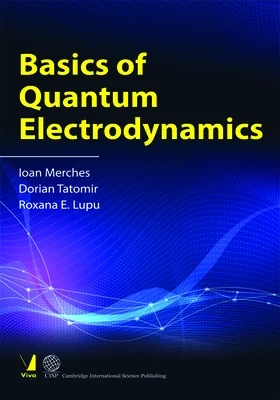Viva Group has been honoured with the Best Publisher Award 2022 by the Delhi State Booksellers & Publishers' Association.

#### Basics of Quantum Electrodynamics## Basics of Quantum Electrodynamics

• By: Ioan Merches, Dorian Tatomir & Roxana E. Lupu

₹1,705.50 ₹1,895.00 Save: ₹189.50 (10%)

• Out of Stock

ISBN: 9789392299315

Bind: Hardback

Year: 2022

Pages: 352

Size: 6 x 9 Inch

Publisher: Cambridge International Science Publishing

Published in India by: Viva Books

Exclusive Distributors: Viva Books

Sales Territory: India, Nepal, Pakistan, Bangladesh, Sri Lanka

Description:

Quantum Field Theory (QFT), sometimes called ”second quantization,” is one of the greatest achievements of the human mind. It is a fact that no modern research, in both physics and any of its diverse branches (condensed matter physics, statistical mechanics, stochastic processes, etc.), can be approached without a good background in QFT. This theory brings together quantum mechanics and relativity, providing a natural framework for describing subatomic phenomena. In particular, the interactions between subatomic particles are intermediated by fields, such as the electromagnetic field. Quantum electrodynamics (QED) is the quantum field theory that describes the interaction of electrically charged particles via electromagnetic fields. This theory has proven spectacularly successful in describing the interaction of light with matter. One of the founding fathers of QED, Richard Feynman, has called it “the jewel of physics for its extremely accurate predictions (the anomalous magnetic moment of the electron, the Lamb shift, etc.).

A text on this subject must present the intimate connection between Quantum Mechanics and QED, at an appropriate level, in two broad steps: first the quantization of the free fields involved, and then the theory of their interactions with applications. The authors’detailed presentation of
the field quantization, the original distribution of the theory, and the study of the most important interaction processes in QED make this text a useful guide for those studying physics at both undergraduate and graduate levels.

Target Audience:

This textbook is a useful guide for those studying physics, at both graduate and undergraduate level.

Contents:

CHAPTER I. GENERAL FIELD THEORY • Basic field equations • Infinitesimal Lorentz Transformation • Transformation of the quantities U(r) in particular cases • Invariance of the Lagrangian density under infinitesimal Lorentz transformation • The energy-momentum tensor of a field • The angular momentum tensor of a field • Symmetry transformations • Phase transformations

CHAPTER II. GENERAL PROBLEMS OF FIELD QUANTIZATION • Necessity of field quantization • Commutation and anticommutation relations. Emission and absorption operators • Commutation relations and the Bose-Einstein statistics  • Anticommutation relations and the Fermi-Dirac statistics • Alternative methods of field quantization • Notations and units in QFT

CHAPTER III. QUANTIZATION OF THE SCALAR FIELD • The Lagrangian formalism • Momentum representation • Momentum, energy and charge of the complex scalar field in momentum representation • Commutators of the free scalar field • Products of operators • Vacuum states. The Fock representation • Wick’s theorems

CHAPTER IV. THE QUANTIZATION OF ELECTROMAGNETIC FIELD • Lagrangian formalism • Momentum representation • Momentum, energy and spin of the electromagnetic field in momentum representation • Commutators of the free electromagnetic field • The indefinite metric formalism • The Lorentz-Fermi condition

CHAPTER V. QUANTIZATION OF THE SPINORIAL FIELD • The Dirac equation and the algebra of gamma matrices • Lagrangian formalism • The free particle in the Dirac theory • Energy, momentum, charge and spin of the free spinorial field in momentum representation • Anticommutators of the free spinorial field • Products of spinorial operators

CHAPTER VI. GENERAL PROBLEMS OF FIELD INTERACTIONS • Generalities • The S-matrix • Choice of the interaction Lagrangian density • The Feynmann-Dyson diagrams • Examples of Feynmann-Dyson diagrams • Transition probability • Scattering cross section

CHAPTER VII. NONDIVERGENT SECOND-ORDER PROCESSES • Transition probability for Compton scattering • Differential cross section for Compton scattering • Electron-positron annihilation • Transition probability for Møller scattering • Møller scattering cross section • Photon-photon scattering with electron-positron pair production • Electron-positron scattering

CHAPTER VIII. DIVERGENT SECOND-ORDER PROCESSES • Self-energy diagram of the electron • Self-energy diagram of the photon The vacuum polarization • Mass and charge renormalization

APPENDIX. DISTRIBUTIONS • Unidimensional delta function • Various representations of the delta function • Some functions related to delta • Functions Dm+ and Dm− • Functions Dm and ˜Dm • Functions D0, ˜D0, D0+, D0− • Functions S, ˜ S, S+, S • Retarded and advanced functions • Causal functions

PROBLEMS with SOLUTIONSĎ

REFERENCES

SUBJECT INDEX

10%

## Vacuum Science, Technology and..

By: Pramod K. Naik

ISBN : 9789392299247

₹ 1,615.50 ₹ 1,795.00

10%

## The Theory of Earthquakes

By: Bogaan Felix Apostol

ISBN : 9789392299339

₹ 1,705.50 ₹ 1,895.00

10%

## Introduction to the Theory of ..

By: Bogaan Felix Apostol

ISBN : 9789392299346

₹ 715.50 ₹ 795.00

10%

## Kinetics and Thermodynamics of..

By: Yurii Kashlev

ISBN : 9789392299322

₹ 1,615.50 ₹ 1,795.00

10%

## Phase Transitions in Solids Un..

By: V. D. Blank and E. I. Estrin

ISBN : 9789392299254

₹ 2,065.50 ₹ 2,295.00

10%

## The Ten Most Beautiful Experim..

By: Ajoy Ghatak

ISBN : 9789392299186

₹ 445.50 ₹ 495.00